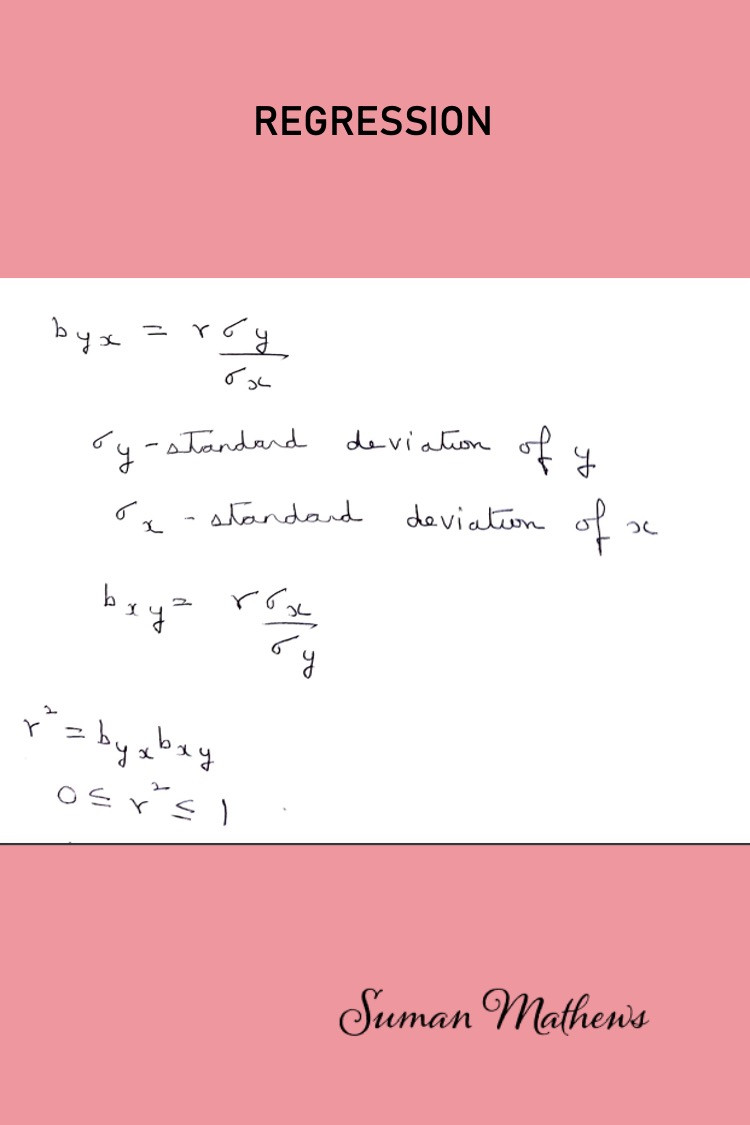Search

# The First Step You Can Take to Understand Regression Analysis

## You will learn what is Regression and the 2 lines of regression.Regression -1

## There are 2 coefficients of Regression , namely the regression coefficient of y on x and the regression coefficient of x on y. The formulas of each is given here.Coefficients of Regression

## You will be introduced to the 2 lines of regression, namely the regression line of y on x, where y is the dependent variable and the regression line of x on y where x is the dependent variable.Regression Equations

## Basically, all the formulas that you need for problem solving are provided in these posts.Regression coefficients in terms of standard deviation